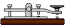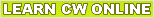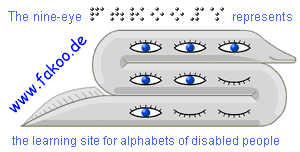fakoo.de/en Alphabets of disabled people

# The Morse Alphabet

Use of the Morse alphabet by Friedrich Clemens Gerke in deafblind people

The Morse alphabet is sometimes used in addition to the Lorm Alphabet (which contains only letters) for the deafblind. The characters are tapped in the palm of theres hand (tactile Morse). Other users are people with limited mobility.

The alphabet invented by Friedrich Clemens Gerke in 1848 is named for the inventor of the first writing telegraph (1837) Samuel Morse.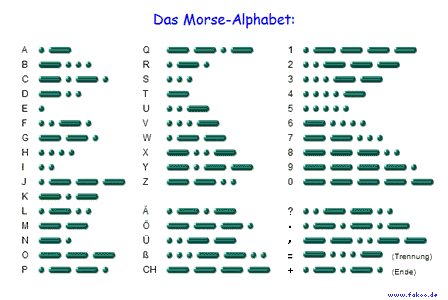``` ---------- Morse Alphabet (Morse code): ---------- A = .- = short long = dit-da B = -... = long short short short = da-dit-dit-dit C = -.-. = long short long short = da-dit-da-dit D = -.. = long short short = da-dit-dit E = . = short = dit F = ..-. = short short long short = dit-dit-da-dit G = --. = long long short = da-da-dit H = .... = short short short short = dit-dit-dit-dit I = .. = short short = dit-dit J = .--- = short long long long = dit-da-da-da K = -.- = long short long = da-dit-da L = .-.. = short long short short = dit-da-dit-dit M = -- = long long = da-da N = -. = long short = da-dit O = --- = long long long = da-da-da P = .--. = short long long short = dit-da-da-dit Q = --.- = long long short long = da-da-dit-da R = .-. = short long short = dit-da-dit S = ... = short short short = dit-dit-dit T = - = long = da U = ..- = short short long = dit-dit-da V = ...- = short short short long = dit-dit-dit-da W = .-- = short long long = dit-da-da X = -..- = long short short long = da-dit-dit-da Y = -.-- = long short long long = da-dit-da-da Z = --.. = long long short short = da-da-dit-dit 0 = ----- = long long long long long = da-da-da-da-da 1 = .---- = short long long long long = dit-da-da-da-da 2 = ..--- = short short long long long = dit-dit-da-da-da 3 = ...-- = short short short long long = dit-dit-dit-da-da 4 = ....- = short short short short long = dit-dit-dit-dit-da 5 = ..... = short short short short short = dit-dit-dit-dit-dit 6 = -.... = long short short short short = da-dit-dit-dit-dit 7 = --... = long long short short short = da-da-dit-dit-dit 8 = ---.. = long long long short short = da-da-da-dit-dit 9 = ----. = long long long long short = da-da-da-da-dit the complete Morse alphabet as a text version to print to the top```

the graphic print versions of the Morse Alphabet   [text version of the Morse Alphabet (new window) ]

the original alphabet by Samuel Morse

### Explanations: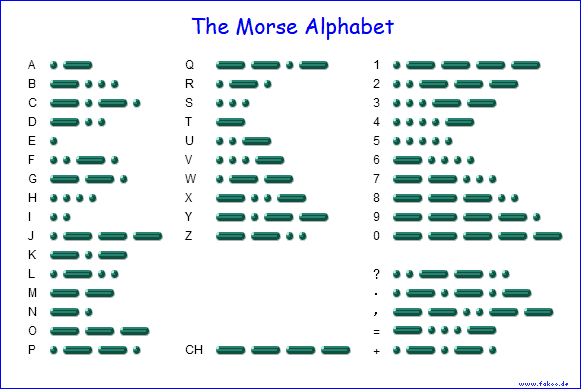##### Further informations:

Inventor of today's Morse code Friedrich Clemens Gerke
Use of the Morse alphabet to control the computer
Morse Codes for Computer Access